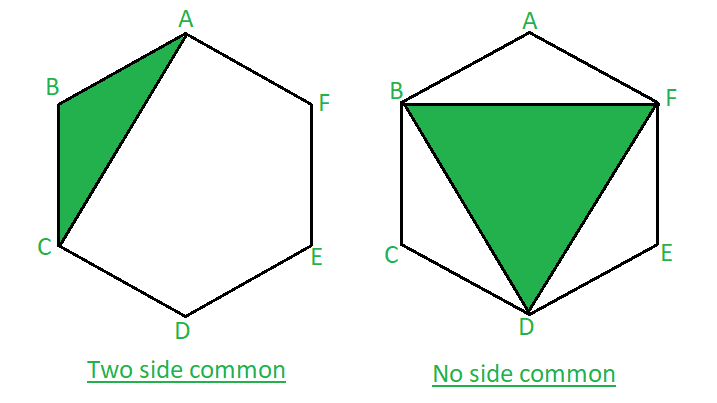GeeksforGeeks App
Open AppBrowser
Continue

# Number of triangles formed by joining vertices of n-sided polygon with two common sides and no common sides

Given N-sided polygon we need to find the total number of triangles formed by joining the vertices of the given polygon with exactly two sides being common and no side being common.
Examples:

Input : N = 6
Output : 6 2
The image below is of a triangle forming inside a Hexagon by joining vertices as shown above.
The triangle formed has two sides (AB and BC) common with that of a polygon. Similarly BC and
CD can make one triangle. With this, we can say that there will be a total of 6 triangles possible
having two sides common with that of a polygon. The second image of a hexagon,
a triangle is formed with no side common with that of a polygon.
There will be just 2 triangles possible, BFD and ACE.Triangle with two side common and no side common of the Hexagon

Number of triangles formed are 6 and 2 with two side common and with no side common respectively.
Input : N = 7
Output : 7 7

Approach :

• To make a triangle two side common with a polygon we will take any one side of a n-sided polygon, take one vertex of the chosen side and join an edge adjacent to the vertex of the other vertex.
• Traversing through each vertex and adjoining an edge adjacent to the vertex of the other vertex ,there will be N number of triangles having two side common.
• Now, to calculate the number of triangles with no side common subtract the total number of triangles with one side common and the total number of triangles with two side from the total number of triangles possible in a polygon.
• Triangles with no common side = Total triangles ( nC3 ) – one side common triangles ( n * ( n – 4 ) – two side common triangles ( n ).
• Thus number of triangles with no common side with the polygon would be equal to n * ( n – 4 ) * ( n – 5 ) / 6.

Note:To calculate the number of triangles having one side common with that of a polygon click here
Below is the implementation of the above approach:

## C++

 `// C++ program to implement``// the above problem``#include ``using` `namespace` `std;` `// Function to find the number of triangles``void` `findTriangles(``int` `n)``{``    ``int` `num = n;` `    ``// print the number of triangles``    ``// having two side common``    ``cout << num << ``" "``;` `    ``// print the number of triangles``    ``// having no side common``    ``cout << num * (num - 4) * (num - 5) / 6;``}` `// Driver code``int` `main()``{``    ``// initialize the number``    ``// of sides of a polygon``    ``int` `n;``    ``n = 6;` `    ``findTriangles(n);` `    ``return` `0;``}`

## Java

 `// Java program to implement``// the above problem``import` `java.io.*;` `class` `GFG``{`  `// Function to find the number of triangles``static` `void` `findTriangles(``int` `n)``{``    ``int` `num = n;` `    ``// print the number of triangles``    ``// having two side common``    ``System.out.print( num + ``" "``);` `    ``// print the number of triangles``    ``// having no side common``    ``System.out.print( num * (num - ``4``) * (num - ``5``) / ``6``);``}` `// Driver code``public` `static` `void` `main (String[] args)``{``    ``// initialize the number``    ``// of sides of a polygon``    ``int` `n;``    ``n = ``6``;` `    ``findTriangles(n);``}``}` `// This code is contributed by anuj_67..`

## Python3

 `# Python3 program to implement``# the above problem` `# Function to find the number of triangles``def` `findTriangles(n):``    ``num ``=` `n``    `  `    ``# print the number of triangles``    ``# having two side common``    ``print``(num, end ``=` `" "``)` `    ``# print the number of triangles``    ``# having no side common``    ``print``(num ``*` `(num ``-` `4``) ``*` `(num ``-` `5``) ``/``/` `6``)` `# Driver code` `# initialize the number``# of sides of a polygon``n ``=` `6``;` `findTriangles(n)` `# This code is contributed by Mohit Kumar`

## C#

 `// C# program to implement``// the above problem``using` `System;` `class` `GFG``{`  `// Function to find the number of triangles``static` `void` `findTriangles(``int` `n)``{``    ``int` `num = n;` `    ``// print the number of triangles``    ``// having two side common``    ``Console.Write( num + ``" "``);` `    ``// print the number of triangles``    ``// having no side common``    ``Console.WriteLine( num * (num - 4) * (num - 5) / 6);``}` `// Driver code``public` `static` `void` `Main ()``{``    ``// initialize the number``    ``// of sides of a polygon``    ``int` `n;``    ``n = 6;` `    ``findTriangles(n);``}``}` `// This code is contributed by anuj_67..`

## Javascript

 ``

Output:

`6 2`

Time Complexity: O(1)

Auxiliary Space: O(1)

My Personal Notes arrow_drop_up